# ICSE solutions for Class 10 Physics chapter 6 - Echoes and Vibrations of Sound [Latest edition]

#### Chapters## Chapter 6: Echoes and Vibrations of Sound

### ICSE solutions for Class 10 Physics Chapter 6 Echoes and Vibrations of SoundShort Answers

What is sound? What causes it to produce?

Define the term Amplitude.

Define the term Frequency (as applied to sound waves)

What are mechanical waves?

Name the unit used to measure the sound level.

Name the subjective property of sound related to its frequency.

Name the subjective property of light related to its wavelength.

State two differences between light waves and sound waves.

How does frequency affect a musical sound?

Can sound waves pass through vacuum?

How is sound perceived by us?

Define sound ranging. Give one use of sound ranging.

What are the ultrasonic waves?

What are infrasonic waves?

Arrange the speeds of sound in gases, solids, and liquids in ascending order.

Point out the waves from the given list, i.e., light, sound, radio waves, ultrasonic waves, and thermal radiation, the energy is propagated by mechanical waves.

Write the approximate value of the speed of sound in air.

Write the approximate value of the speed of sound in water.

Write the approximate value of the speed of sound in iron.

Two astronauts cannot hear each other on the moon. Why?

Name the factors which determine the loudness of a note.

Name the factors which determine the pitch of a note.

Name the factors which determine the quality of a note.

How can one distinguish the sounds of two musical instruments even if they are of the same pitch and same loudness?

If the amplitude of a wave is doubled, what will be the effect on its loudness?

How do you account for the fact that two strings can be used to give notes of the same pitch and loudness but of different quality?

The same musical note when played on a piano and when played on a flute sounds different.

Name three characteristics of a musical sound.

Name one main factor on which each character such as intensity, pitch, and quality of musical sound depends.

When a gun is fired at a distance, why is it that the flash is seen almost instantaneously while the sound is heard a little later?

Mention two properties of a wave, one property which varies and the other which remains constant when the wave passes from one medium to another.

Name one property of waves that do not change when the wave passes from one medium to another.

A bucket kept under a running tap is getting filled with water. A person sitting at a distance is able to get an idea when the bucket is about to be filled.
(i) What change takes place in the sound to give this idea?
(ii) What causes the change in the sound?

Which characteristics of sound will change if there is a change in its amplitude and its waveform.

What is the range of audibility?

What is meant by an echo?

What is the condition necessary for an echo to be heard distinctly?

What is meant by echo depth sounding?

Why will an echo not be heard when the distance between the source of the sound and the reflecting surface is 10 m?

State two applications of echo.

What is sonar? State the principle on which it is based.

How are bats able to fly in the dark?

What do you understand by the ‘Echolocation system of Dolphins’?

What are fishing boats?

How does the medium affect the amplitude of free vibrations of a body?

State two factors on which the natural frequency of a body depends.

A metallic blade is made to vibrate at its one end by keeping its other end clamped. The vibrations of the blade die away after some time. Explain, how you lower the frequency of the vibration of the blade?

What are damped vibrations?

Give one example of damped vibrations

What are forced vibrations?

Give two examples of forced vibrations.

What do you mean by resonance? When does resonance occur?

Explain why does a wine glass starts rattling, when a note of some particular frequency is struck by a piano?

When acoustic resonance takes place, a loud sound is heard. Why does this happen? Explain.

Explain why stringed musical instruments, like the guitar, are provided with a hollow box.

State two ways in which resonance differs from forced vibrations.

The rearview mirror of a motorbike starts vibrating violently at some particular speed of the motorbike. What is the name of the phenomenon taking place? Why does the happen?

The rearview mirror of a motorbike starts vibrating violently at some particular speed of the motorbike, what could be done to stop the violent vibrations.

When a troop crosses a suspension bridge the soldiers are asked to break steps. Explain the reason.

Explain why are soldiers asked to walk out of step while crossing bridges?

A cork piece is floating on the surface of the water in a pond. A piece of pebble is dropped into the water. What will be your observation?

Explain why does the rear mirror of a motorbike start Vibrating Violently, at some particular speed of motorbike?

Explain a tuning fork (vibrating) is held close to ear. One hears a faint sound. The same vibrating tuning fork is placed on table, such that its handle is in contact with table, one hears a loud sound.

With which of the following frequencies does a tuning fork of frequency 256 Hz resonate? 288 Hz, 314 Hz, 333 Hz, 512 Hz.

A vibrating tuning fork is placed over the mouth of a burette filled with water. The tap is opened and the water level gradually falls. It is observed that the sound becomes the loudest for a particular length of the air column. What is the name of the phenomenon taking place when this happens? Why does the sound become the loudest?

When a tuning fork, struck by a rubber pad, is held over a length of the air column in a tube, it produces a loud sound for a fixed length of the air column. Name the above phenomenon. How does the frequency of the loud sound compare with that of the tuning fork? State the unit for measuring loudness.

Explain a person walking past a railway line, in the middle of night hears a ringing sound along with the sound of his footsteps.

Name three factors on which the frequency of vibration of a stretched string depends.

What adjustments would you make for tuning a stringed instrument for it to emit a note of a desired frequency?

A string stretched between its ends is made to vibrate by placing the stem of a vibrating tuning fork at its one end. State three ways how you will increase the frequency of note produced by the string.

Explain why strings of different thicknesses are provided on a stringed instrument.

Explain Why are stringed musical instruments provided with large sound boxes?

Explain why strings of different thicknesses are provided on a stringed instrument.

The speed of sound is more in hydrogen than in nitrogen. Is this statement true? Give a reason for your answer.

How is it possible to detect the filling of a pitcher under a tap by hearing its sound at a distance?

Three musical instruments give out notes at the frequencies listed below. Flute: 400 Hz; Guitar: 200 Hz; Trumpet: 500 Hz. Which one of these has the highest pitch?

How do you account for the fact that two strings can be used to give notes of the same pitch and loudness but of different quality?

What change do you expect in the characteristics of a musical sound if
(i) Its frequency is increased,
(ii) Its amplitude is increased?

A type of electromagnetic wave has a wavelength of 50 Å. Name the wave and state one use of this type of wave.

Name the type of waves that are used for sound ranging. Why are radio waves not audible to us?

What is musical sound? Give an example.

What is the noise? Give an example.

### ICSE solutions for Class 10 Physics Chapter 6 Echoes and Vibrations of SoundLong Answers

When a sound is made from a distance of 18 m, in front of a tall building, it is found to get repeated again. Which phenomenon is associated with this observation? Give a brief explanation of this phenomenon?

Why is an echo not heard when the distance between the source of sound and reflection body is less than 10 m?

What do you understand by free (or natural) vibrations?

What do you understand by forced vibrations?

The rearview mirror of a motorbike starts vibrating violently at some particular speed of the motorbike, what could be done to stop the violent vibrations.

What are the factors that affect the frequency of a vibrating string and how do they affect the frequency?

What is meant by the ‘pitch’ of a note? Does it depend on the loudness or ‘quality’ of the ‘note’? How is pitch ‘related’ with frequency?

What is meant by the ‘loudness’ of a sound? How is it related to the intensity?

### ICSE solutions for Class 10 Physics Chapter 6 Echoes and Vibrations of SoundFigure Based Short Answers

Figure Based Short Answers | Q 1

How does the wave pattern of a loud note differ from a soft note? Draw a diagram.

Figure Based Short Answers | Q 2

Draw a diagram to show the waveforms of a pure note and a musical note, of the same pitch and same loudness.

Figure Based Short Answers | Q 3

Draw a diagram representing a wave of amplitude 4 cm and wavelength 2 m. If the frequency of the wave is 150 Hz, calculate its velocity.

Figure Based Short Answers | Q 4

What do you understand by free vibrations of a body? Draw a displacement-time graph to represent them. Given one example.

Figure Based Short Answers | Q 5

Draw a displacement time graph to illustrate damped vibrations.

Figure Based Short Answers | Q 6

Two musical notes of the same pitch and loudness are played on a violin and a piano. Their waveforms are as shown in the figure below: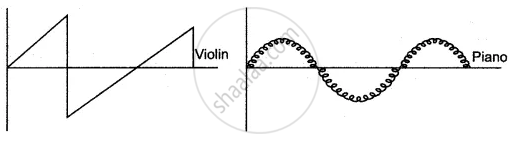Figure Based Short Answers | Q 7

The frequency of the stretched string of a fixed length is f when it is plucked in the middle. Where should it be plucked so that string vibrates with frequency (i) 2f, (ii) 3f?
Draw diagrams to show these vibrations and name them.

Figure Based Short Answers | Q 8

In what respect does waveforms of noise and a musical note differ. Draw diagrams to illustrate your answer.

### ICSE solutions for Class 10 Physics Chapter 6 Echoes and Vibrations of SoundFigure Based Long Answers

Figure Based Long Answers | Q 1

A cannon is fired at A (see diagram). An observer at B hears two sounds. The first sound is heard after 1 second and the second 3s after the observer sees the flash. (Velocity of sound = 340 ms−1)
(i) Why does the observer see the flash before he hears the sound?
(ii) Calculate the distance x between the observer and the wall.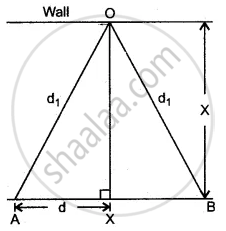Figure Based Long Answers | Q 2

An observer X is on an island 5500 m from a vertical cliff on the shore. A ship Y is anchored between the island and the Cliff. A blast on the ship’s siren is heard twice by X, the interval being 5s. [Velocity of sound = 330 ms−1]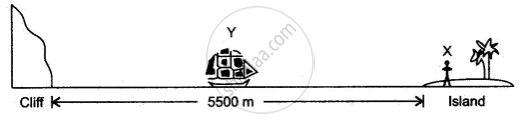(i) Find the distance of the ship from the island.
(i) Find the distance of the cliff from the ship.

Figure Based Long Answers | Q 3

The following diagram shows the displacement – time graph of the vibrating body.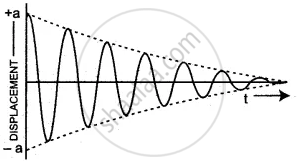(i) name the kind of vibrations
(ii) Give one example of such vibrations
(iii) why is the amplitude of vibrations gradually decreasing?
(iv) what happens to the vibrations of the body after some time?

Figure Based Long Answers | Q 4

Explain free and forced vibrations. Give an experimental arrangement to illustrate the phenomenon of resonance.

Figure Based Long Answers | Q 5

Describe a simple experiment to illustrate the phenomenon of resonance and explain it.

Figure Based Long Answers | Q 6

The diagram below shows three ways in which the string of an instrument can vibrate.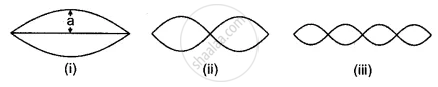(i) Which of the above diagrams shows the fundamental note?
(ii) Which has the frequency 4 times that of the fundamental?
(iii) State the ratio between the frequency of the first and second.

Figure Based Long Answers | Q 7

A 0.6 m long stretched wire is made to vibrate in two different modes as shown in figure

(a)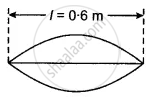(b)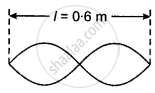(i) If the frequency of the note produced in (b) is n, what is the frequency in case (a)?
(ii) In which case (a) or (b), is the note louder? Give reasons.
(iii) In which case is the pitch higher? Give reason.

Figure Based Long Answers | Q 8

The adjacent diagram shows three different modes of vibrations P, Q and R of the same string.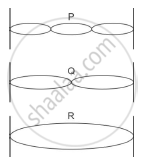(i) Which vibration will produce a louder sound and why?
(ii) The sound of which string will have maximum shrillness?
(iii) State the ratio of wavelengths of P and R.

Short Numerical

### ICSE solutions for Class 10 Physics Chapter 6 Echoes and Vibrations of SoundShort Numerical

Short Numerical | Q 1

A radio signal, sent towards the surface of the moon, is received in 2.5 seconds. If the speed of the radio waves is 3 × 108 ms−1, find the distance of the moon from the earth.

Short Numerical | Q 2

A sound made on the surface of a lake takes 3 s to reach a boatman. How much time will it take to reach a diver inside the water at the same depth?
Velocity of sound in air = 330 ms1
Velocity of sound in water = 1450 ms1

Short Numerical | Q 3

Two waves of the same pitch have their amplitudes in the ratio 2 : 3.
(i) What will be the ratio of their loudness?
(ii) What will be the ratio of their frequencies?

Short Numerical | Q 4

The smoke from the gun barrel is seen 2 seconds before the explosion is heard. If the speed of sound in air is 340 ms−1, calculate the distance of the observer from the gun. State the approximation used.

Short Numerical | Q 5

A boy standing in front of a wall at a distance 17 m produces 10 claps per second. He notices that the sound of his clapping coincides with the echo. Echo is heard only once when clapping is stopped. Calculate the speed of sound.

Short Numerical | Q 6

An observer standing in front of a vertical wall claps 10 times one second. He adjusts his distance from the wall in such a way that the second of his clapping coincides with the echo. This happens when bis distance from the wall is 17.5m. What is the velocity of sound heard by him?

Short Numerical | Q 7

A person fires a gun while standing at a distance of 850 m from a building. If the speed of sound is 350 ms, calculate the time in which the person hears an echo of the gun flte.

Short Numerical | Q 8

A boy stands 66.4 m in front of a high wall and then blows a whistle. Calculate the time interval when he hears an echo. (Speed of sound = 332m/s).

Short Numerical | Q 9

A ship which is stationary at a distance of 680 m from the shore, sends a signal to the coast. Its echo is heard in 4s. Find the velocity of sound.

Short Numerical | Q 10

An observer stands at a distance of 850 m from a cliff and fires a gun. After what time gap will he hear the echo? If sound travels at a speed of 350 m/sec., in air.

Short Numerical | Q 11

The wavelength and frequency of the sound wave in a certain medium is 40 cm and 835 Hz respectively. In the same medium if another wave has a wavelength equal to 32 cm, calculate its frequency.

Short Numerical | Q 12

A pendulum has a frequency of 5 vibrations per second. An observer starts the pendulum and fires a gun simultaneously. He hears echo from the cliff after 8 vibrations of the pendulum. If the velocity of sound in air is 340 m s-1, find the distance between the cliff and the observer.

Short Numerical | Q 13

A radar sends a signal to an aircraft at a distance of 30 km away and receives it back after 2 x 10-4 seconds. What is the speed of the signal?

Short Numerical | Q 14

An observer stands at a certain distance away from a cliff and produces a loud sound. He hears the echo of the sound after 1.8 s. Calculate the distance between the cliff and the observer if the velocity of sound in air is 340 m−1.

Short Numerical | Q 15

A man standing between two cliffs produces a sound and hears two successive echoes at intervals of 3s and 4s respectively. Calculate the distance between the two cliffs. The speed of sound in the air is 330 ms−1.

Short Numerical | Q 16

An instrument is able to detect the reflected waves from an enemy aeroplane, after a time interval of 0.02 milliseconds. If the velocity of the waves is 3 × 108 ms−1, calculate the distance of the plane from the radar.

Long Numerical

### ICSE solutions for Class 10 Physics Chapter 6 Echoes and Vibrations of SoundLong Numerical

Long Numerical | Q 1

A man stands in between two parallel cliffs and blows a whistle. He hears first echo after 0.6s and second echo after 2.4s. Calculate the distance between cliffs.
[Speed of sound = 336 m/s]

Long Numerical | Q 2

A boy stands in from of a cliff on the other side of a river. He fires a gun and hears an echo after 6 seconds. The boy then moves backward by 170 m and again fires the gun. He hears an echo after 7 seconds. Calculate
(i) width of river,
(ii) speed of sound.

Long Numerical | Q 3

A person standing between two vertical cliffs and is 640 m away from the nearest cliff. He shouted and heard the first echo after 45s and the second echo after further 3s. Calculate the speed of sound in air and the distance between the cliffs.

Long Numerical | Q 4

A man standing in front of a vertical cliff fires a gun. He hears an echo after 35s. On moving close to the cliff by 82.5 m he fires again. This time he hears the echo after 2.55s. Calculate the speed of sound, distance, and the initial position of man from the cliff.

Long Numerical | Q 5

Waves produced on the surface of the water are formed to move with a velocity of 24 ms−1. If the wavelength of these waves equals 20 cm. find the
(i) frequency (no. of waves produced per second)
(ii) the time period (i.e. the time required to produce one wave) for these waves.

Long Numerical | Q 6

A man standing 25 m away from a wall produces a sound and receives the reflected sound. (a) Calculate the time after which he receives the reflected sound if the speed of the sound in air is 350 m s-1. (b) will the man be able to hear a distinct echo? Explain the answer.

Long Numerical | Q 7

(i) A man stands at a distance of 68 m from a cliff and fires a gun. After what time interval will he hear the echo, if the speed of sound in air is 340 ms−1?
(ii) If the man had been standing at a distance of 12 m from the cliff would he have heard a clear echo?

Long Numerical | Q 8

A sound wavelength of 0.332 m has a time period of 10−3 s. If the time period is decreased to 10−4 s, calculate the wavelength and frequency of the new wave.

Long Numerical | Q 9

A sound wavelength of 0.332 m has a time period of 10−3 s. If the time period is decreased to 10−4 s, calculate the wavelength and frequency of the new wave.

## Chapter 6: Echoes and Vibrations of Sound## ICSE solutions for Class 10 Physics chapter 6 - Echoes and Vibrations of Sound

ICSE solutions for Class 10 Physics chapter 6 (Echoes and Vibrations of Sound) include all questions with solution and detail explanation. This will clear students doubts about any question and improve application skills while preparing for board exams. The detailed, step-by-step solutions will help you understand the concepts better and clear your confusions, if any. Shaalaa.com has the CISCE Class 10 Physics solutions in a manner that help students grasp basic concepts better and faster.

Further, we at Shaalaa.com provide such solutions so that students can prepare for written exams. ICSE textbook solutions can be a core help for self-study and acts as a perfect self-help guidance for students.

Concepts covered in Class 10 Physics chapter 6 Echoes and Vibrations of Sound are Properties of Sounds, Sound, Characteristics of a Sound Wave, Reflection of Sound, Natural Vibrations, Echoes, Damped Vibrations, Forced Vibrations, Loudness and Intensity, Resonance, Demonstration of Resonance, Pitch (or shrillness) and frequency, Audibility and Range, Quality (Or Timbre) and Wave Form, Noise Pollution, Noise and Music, Sound (Numerical), Difference Between the Sound and Light Waves.

Using ICSE Class 10 solutions Echoes and Vibrations of Sound exercise by students are an easy way to prepare for the exams, as they involve solutions arranged chapter-wise also page wise. The questions involved in ICSE Solutions are important questions that can be asked in the final exam. Maximum students of CISCE Class 10 prefer ICSE Textbook Solutions to score more in exam.

Get the free view of chapter 6 Echoes and Vibrations of Sound Class 10 extra questions for Class 10 Physics and can use Shaalaa.com to keep it handy for your exam preparation